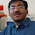Apr 11, 2011

Smallest Number in Decreasing Sequence

Source: Quantnet Forums

Problem:
You pick random numbers between 0 and 1 (uniformly at random) x1, x2, x3.. as long as they keep decreasing x1 > x2 > x3 > ...

What is the expected value of the smallest number you pick?

Update (26-05-2011):
Solution: Posted by Gaurav Sinha (chera, IITK 1996 Graduate, Indian Revenue Service) in comments!
Interesting approach (although wrong) by Gautam Kamath (EE, Senior Undergraduate, IIT Bombay) in comments - Corrected by me!

1.is it 1/3 ?

2.What does it mean by between 0 and 1 if there is a constraint that the subsequent picks are to be less than that of the previous one?

If you choose x in the first pick then the second one is U(0,x) right? If this is the case I am assuming the expected value of last random number chosen is needed.

In this case isn't it just the product of iid uniformly distributed random variables which by independence has an expectation of 1/2^n?

3.got answer 3-e where e is exponential constant.

define f(x) as the conditional expected value given that the last value seen is x.

we want to find f(1).

given that last value seen is x,
there are two cases:

case 1: next value >= x, in this case the sequence stops and the value you pick is x.

case 2: next value < x. In this case , the sequence continues. And the conditional expected value is
= integral from z=0 to z=x, f(z)dz/x.

probability of case 1 is 1-x,
probability of case 2 is x.

so, f(x)= (1-x).x + x. (integral from z=0 to z=x, f(z)dz/x).

differentiating w.r.t. x,
df(x)/dx=1-2x+f(x)
also f(0)=0
we solve to get f(x)= 2x+1-e^x.
so f(1)=3-e.

4.Sorry. Misunderstood the question initially. so there is no constraint but rather a stopping condition.

But nice question and quite an impressive solution by chera.

5.BTW Can you mention the source.

6.7.Let us consider that the smallest number lies in the region dx. This number would be smallest only if it is in the second last position of the sequence and all the other numbers are greater than x.
Let f(x) be the probability of the smallest number belonging in the region dx.
Then f(x) = dx*(1-x) + dx*(1-x)^2/1! + dx*(1-x)^3/2!+....
This is so because probability of selecting a number in dx area is dx and then all other numbers should lie in the region (x,1). Also the last number can be any number among the n numbers that we have selected. But for the remaining n-1 numbers there is only 1 decreasing sequence possible so we need to divide by (n-1)!.
This gives us f(x) = dx*xe^x
So expected value is integration dx*x^2*e^x from 0 to 1.
This gives us final answer as 3-e.

8.Suppose x is the smallest number. The probability of this happening is given by the following idea:
Sum (over all n) of [Probability that x was chosen at the nth step]
Now,the probability that x was chosen at the nth step implies:
1) All previous numbers > n (corresponds to (1-x)^n)
2) They are arranged in descending order (corresponds to 1/(n!))

Hence the probability density is given by summation((1-x)^n/(n!)) which is exp(1-x)-1.
Now simply integrate x*(exp(1-x)-1) from 0 to 1 to get the desired value of expectation
The value I calculated was e-2.5 = 0.21828

9.@chera: Thanks for the solution

@Gautam:
Some mistakes in your solution

Now,the probability that x was chosen at the nth step implies:
1) All previous numbers > x (corresponds to (1-x)^(n-1))
2) They are arranged in descending order (corresponds to 1/(n-1!))
3) The element after x is > x, so, probability (1-x)

Hence the probability density is given by summation((1-x)^n/(n-1!)) which is (1-x)*exp(1-x).
Now simply integrate x*(1-x)*exp(1-x) from 0 to 1 to get the desired value of expectation

Applying Integration by parts twice, we get 3-e. Solved. :)

@yash.. What do you mean by region "dx"? Can you make your solution mathematically precise please? Thanks.

Fraction Brainteaser

Source: Sent to me by Gaurav Sinha Problem: Siddhant writes a Maths test and correctly answers 5 out of 6 Arithmetic questions and 20...